# Stability Analysis of Control Systems Notes | Study Electrical Engineering SSC JE (Technical) - Electrical Engineering (EE)

## Electrical Engineering (EE): Stability Analysis of Control Systems Notes | Study Electrical Engineering SSC JE (Technical) - Electrical Engineering (EE)

The document Stability Analysis of Control Systems Notes | Study Electrical Engineering SSC JE (Technical) - Electrical Engineering (EE) is a part of the Electrical Engineering (EE) Course Electrical Engineering SSC JE (Technical).
All you need of Electrical Engineering (EE) at this link: Electrical Engineering (EE)

## STABILITY ANALYSIS OF CONTROL SYSTEMS

• Roughly speaking, stability in a system implies that small changes in the system input, in initial conditions or in system parameters, do not result in large changes in system output.
• A linear time invariant system is stable if the following two notions of system stability are satisfied:

⇒ When the system is excited by bounded input, the output is bounded.
⇒ In the ad to absence of the input, output tends towards zero (the equilibrium state of the system) irrespective of initial conditions. This stability concept is known as asymptotic stability.

•  The first notion concerns a system under the influence of an input. Clearly, if a system is subjected to an unbounded input and produces an unbound response, nothing can be said about its stability. But if it is subject to a bounded input and produces an unbounded output, it is by definition, unstable.
• Actually the output of an unstable system may increase to a certain extent and then the systemmay breakdown or become non-linear after  the output exceeds a certain magnitude, so that the linear mathematical model no longer applies.

⇒ The nature of response terms contributed by all possible types of roots is given below:

• If all the roots of the characteristic equation have negative real parts, then the impulse response is bounded and eventually decrease to zero. Hence given system is absolutely stable.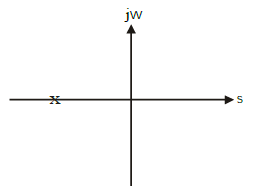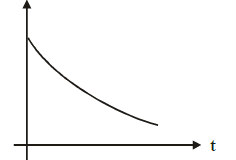• If any root of the characteristic equation has positive real part, then system is unstable.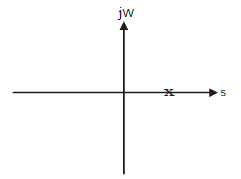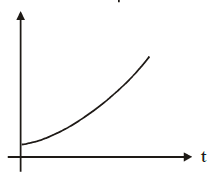• if the characteristic equation has repeated roots on the jw-axis, then system is unstable.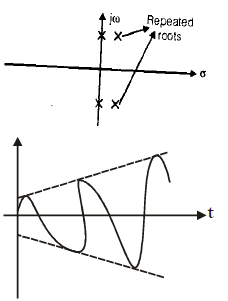• single root at origin or non -multiple roots on jw-axis contribute response term which are constant amplitude or constant amplitude oscillations. This type of system is marginally stable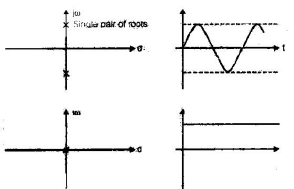ROUTH HURWITZ STABILITY CRITERION

• A. Hurwitz E. J. Routh developed the method of investigating the sufficient conditions of stability of a system.
• The Hurwitz criterion is in terms of determinants and Routh criterion is in terms of array formulation, which is more convenient to handle.
• In this section we will see only Routh Criterion.

Routh Stability Criterion

• This criterion is based on ordering the coefficients of the characteristic equation into array called Routh array as given below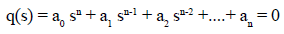Routh Array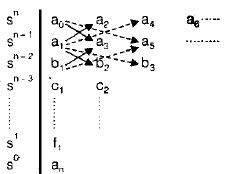• The coefficients b1, b2...are evaluated as follows: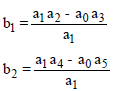• This method is continued till we get a zero arraas the last coefficient in the third row, in the similar way the coefficients of 4th, 5th.....nth, (n + 1)th rows are evaluated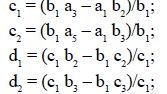• The Routh Stability criterion is stated as follows: For a system to be stable, it is necessary and sufficient that each term of first column of Routh array of its characteristic equation be positive (if a0 > 0). If this condition is not met, the system is unstable and number of sign changes of the terms of the first column of the Routh array corresponds to the number of roots of the characteristic equation in the right half of s-plane.

Note:

⇒ In the process of generating Routh array, the missing terms are regarded as zero. Also all the elements of any row can be divided by a positive constant during the process to simplify the computational work.
⇒ It is be noted that Routh array gives only the number of roots in the right half of splane. If gives no information regarding the values of the roots and also does not distinguish between real and complex roots.
⇒ If there is any missing term or any negative coefficient associate then system will not be stable

Special Cases
case

• If a first column term in any row is zero, but the remaining terms are not zero or there is no remaining term then the zero is replaced with a small positive number Î and rest of the array is evaluated.
• Example: consider the following polynomial

s3 + 2s2 + s + 2 = 0

The array of coefficients is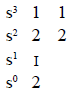• It the sign of the coefficient above the zero ( Î ) is same as that below it; it indicates that there are a pair of imaginary roots. Actually equations (6.1) has two roots at s = ± j.
• If however, the sign of the coefficient above the zero ( Î ) is opposite that below it, indicates there is one sign change.
•  Example
Consider the following equation
s3 – 3s + 2 = (s-1)2 (s + 2) = 0
the array of coefficients is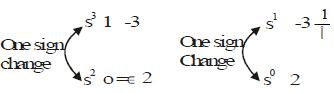• By looking at the polynomial also we can say that this is an unstable system as there is a missing term (s2) and also the coefficient of s is negative.
• Coming at the Routh array as there is different sign on the both sides of zero ( Î ) and also there is one sign change from (-3-2/ Î to 2). So from Routh array there are total two sign change and two roots on right hand side, which agrees with the factored form of characteristic equation

case-2

• when all the elements in any one row of the Routh array are zero. This condition indicates that there are symmetrically located roots in the s-plane (pair of real roots with opposite sign and /or pair of conjugate roots on the imaginary axis and/or complex conjugate roots forming quadrates in the s=phane.)
• The polynomial whose coefficients are the elements of the row just above the row of zeros in the routh array is called auxiliary polynomial.
• Because of a zero row in the array, the routh's test breaks down. This situation is overcome by replacing the row of zeros in the Routh array by a row of coefficients of the polynomial generated by taking the first derivative of the auxiliary polynomial

RELATIVE STABILITY ANALYSIS

• Once the system is shown to be stable, we proceed to determine its relative stability quantitatively by finding the settling time of the dominant roots of its characteristic equation.
• The settling time being proportional to the real part of the dominant roots. the relative stability can be specified by requiring that all the roots of the characteristic equation be more negative than a certain value i.e. all the roots of the characteristic equation of the system under study is then modified by shifting the origin of the splane to s = – s , i.e. by the substitution s = z – s
• It the new characteristic equation in z-satisfies the Routh criterion, it implies that all the roots of the original characteristic equation are more negative than – s 1.
The document Stability Analysis of Control Systems Notes | Study Electrical Engineering SSC JE (Technical) - Electrical Engineering (EE) is a part of the Electrical Engineering (EE) Course Electrical Engineering SSC JE (Technical).
All you need of Electrical Engineering (EE) at this link: Electrical Engineering (EE)Use Code STAYHOME200 and get INR 200 additional OFF

## Electrical Engineering SSC JE (Technical)

2 videos|65 docs|37 tests

Track your progress, build streaks, highlight & save important lessons and more!

,

,

,

,

,

,

,

,

,

,

,

,

,

,

,

,

,

,

,

,

,

;